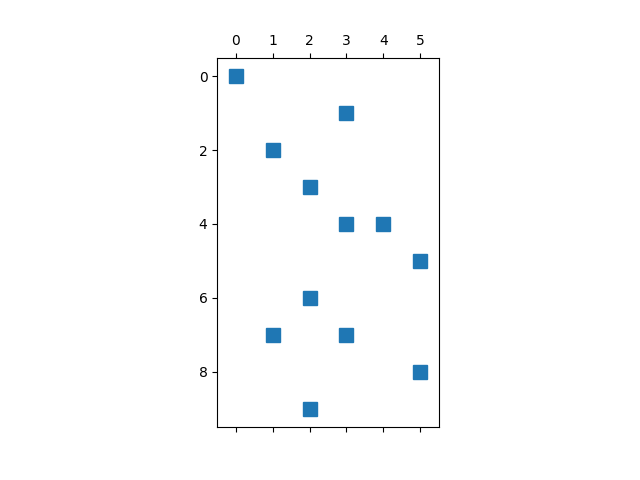# Sparse Inputs¶

We sometimes meet with problems where the $$N × p$$ input matrix $$X$$ is extremely sparse, i.e., many entries in $$X$$ have zero values. A notable example comes from document classification: aiming to assign classes to a document, making it easier to manage for publishers and news sites. The input variables for characterizing documents are generated from a so called "bag-of-words" model. In this model, each variable is scored for the presence of each of the words in the entire dictionary under consideration. Since most words are absent, the input variables for each document is mostly zero, and so the entire matrix is mostly zero.

## Example¶

We create a sparse matrix as our example:

from time import time
from abess import LinearRegression
from scipy.sparse import coo_matrix
import numpy as np
import matplotlib.pyplot as plt

row = np.array([0, 1, 2, 3, 4, 4, 5, 6, 7, 7, 8, 9])
col = np.array([0, 3, 1, 2, 4, 3, 5, 2, 3, 1, 5, 2])
data = np.array([4, 5, 7, 9, 1, 23, 4, 5, 6, 8, 77, 100])
x = coo_matrix((data, (row, col)))


And visualize the sparsity pattern via:

plt.spy(x)
plt.show()## Usage: sparse matrix¶

The sparse matrix can be directly used in abess pacakages. We just need to set argument sparse_matrix = True. Note that if the input matrix is not sparse matrix, the program would automatically transfer it into the sparse one, so this argument can also make some improvement.

coef = np.array([1, 1, 1, 0, 0, 0])
y = x.dot(coef)
model = LinearRegression()
model.fit(x, y, sparse_matrix=True)

print("real coef: \n", coef)
print("pred coef: \n", model.coef_)

real coef:
[1 1 1 0 0 0]
pred coef:
[1. 1. 1. 0. 0. 0.]


## Sparse v.s. Dense: runtime comparsion¶

We compare the runtime when the input matrix is dense matrix:

t = time()
model.fit(x.toarray(), y)
print("dense matrix:  ", time() - t)

t = time()
model.fit(x, y, sparse_matrix=True)
print("sparse matrix:  ", time() - t)

dense matrix:   0.0007922649383544922
sparse matrix:   0.0010333061218261719


From the comparison, we see that the time required by sparse matrix is smaller, and this sould be more visible when the sparse imput matrix is large. Hence, we suggest to assign a sparse matrix to abess when the input matrix have a lot of zero entries.

The abess R package also supports sparse matrix. For R tutorial, please view https://abess-team.github.io/abess/articles/v09-fasterSetting.html

Total running time of the script: ( 0 minutes 0.119 seconds)

Gallery generated by Sphinx-Gallery